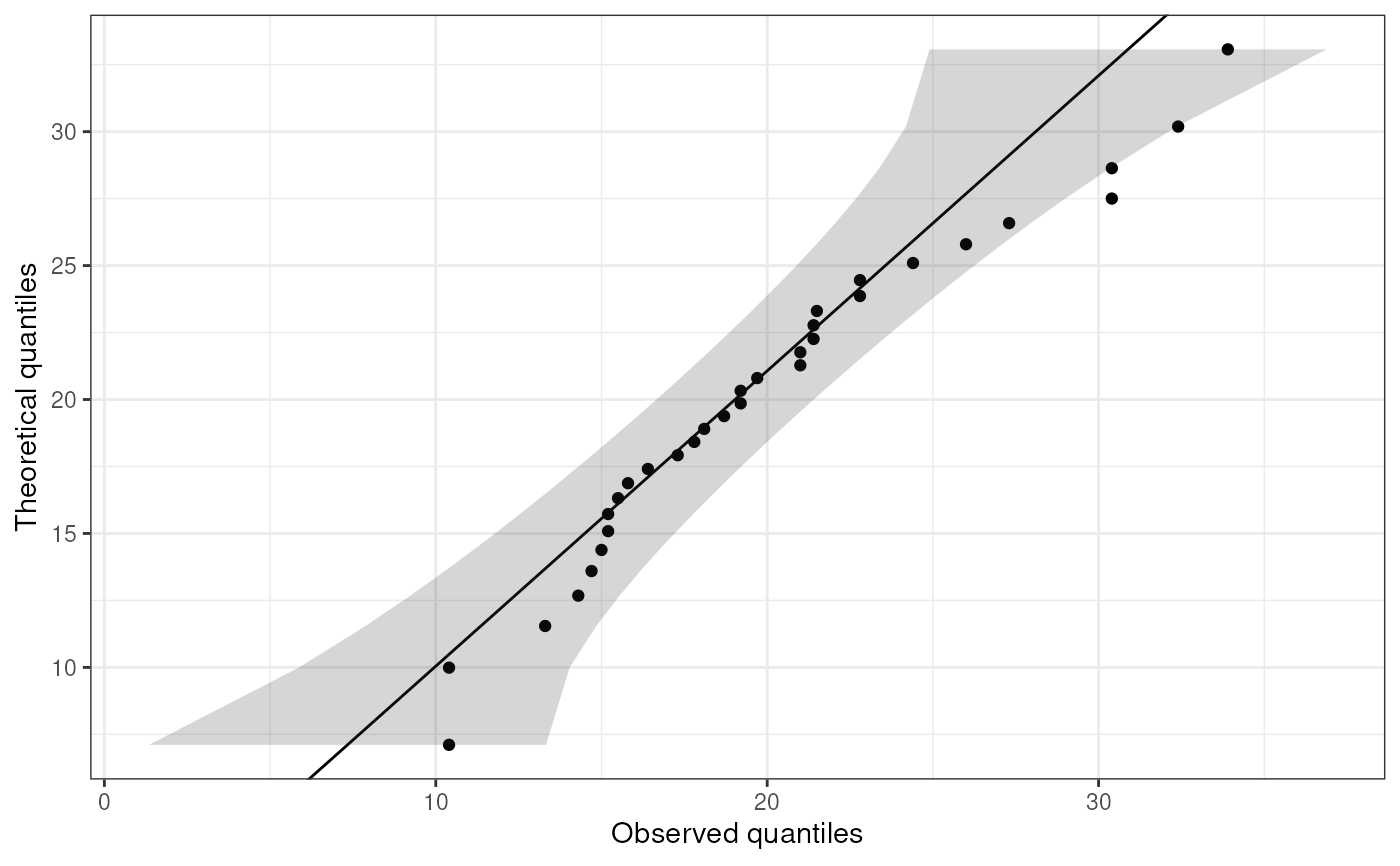This function creates a qq-plot with a confidence interval.

ggqq(
x,
distribution = "norm",
...,
ci = TRUE,
line.estimate = NULL,
conf.level = 0.95,
sampleSizeOverride = NULL,
observedOnX = TRUE,
scaleExpected = TRUE,
theoryLab = "Theoretical quantiles",
observeLab = "Observed quantiles",
theme = ggplot2::theme_bw()
)

## Arguments

x

A vector containing the values to plot.

distribution

The distribution to (a 'd' and 'q' are prepended, and the resulting functions are used, e.g. dnorm and qnorm for the normal curve).

...

Any additional arguments are passed to the quantile function (e.g. qnorm). Because of these dots, any following arguments must be named explicitly.

ci

Whether to show the confidence interval.

line.estimate

Whether to show the line showing the match with the specified distribution (e.g. the normal distribution).

conf.level

THe confidence of the confidence leven arround the estimate for the specified distribtion.

sampleSizeOverride

It can be desirable to get the confidence intervals for a different sample size (when the sample size is very large, for example, such as when this plot is generated by the function normalityAssessment). That different sample size can be specified here.

observedOnX

Whether to plot the observed values (if TRUE) or the theoretically expected values (if FALSE) on the X axis. The other is plotted on the Y axis.

scaleExpected

Whether the scale the expected values to match the scale of the variable. This option is provided to be able to mimic SPSS' Q-Q plots.

theoryLab

The label for the theoretically expected values (on the Y axis by default).

observeLab

The label for the observed values (on the Y axis by default).

theme

The theme to use.

## Value

A ggplot plot is returned.

## Details

This is strongly based on the answer by user Floo0 to a Stack Overflow question at Stack Exchange (see https://stackoverflow.com/questions/4357031/qqnorm-and-qqline-in-ggplot2/27191036#27191036), also posted at GitHub (see https://gist.github.com/rentrop/d39a8406ad8af2a1066c). That code is in turn based on the qqPlot() function from the car package.

## Author

John Fox and Floo0; implemented in this package (and tweaked a bit) by Gjalt-Jorn Peters.

Maintainer: Gjalt-Jorn Peters gjalt-jorn@userfriendlyscience.com

## Examples


ggqq(mtcars\$mpg);